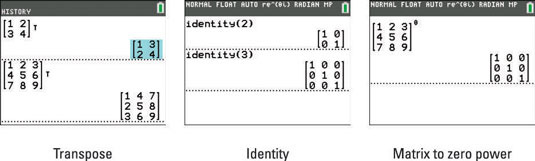##### TI-84 Plus CE Graphing Calculator For Dummies, 3rd EditionQuite a few operations are unique to matrices. All the matrix-specific operations on the TI-84 Plus calculator are found by accessing the MATRX MATH Operations menu (see the first two screens). You access this menu by pressing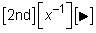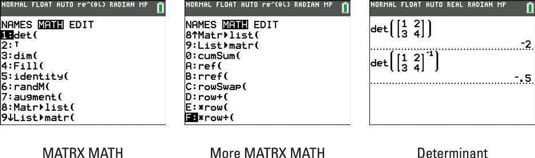The determinant is used to perform all kinds of matrix operations, so the determinant is a good place to start. When finding the determinant of a matrix, the matrix must be square (number of rows = number of columns). If it isn’t, you get the ERROR: INVALID DIMENSION error message.

To evaluate the determinant of a matrix, follow these steps:

1. If necessary, press [2nd][MODE] to access the Home screen.

2. To select the det( command from the MATRX MATH menu, press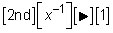3. Enter the matrix.

Press [ALPHA][ZOOM] to create a matrix from scratch, or press [2nd][x–1] to access a stored matrix.

4. Press [ENTER] to evaluate the determinant.

This procedure is illustrated in the third screen.

There are a few other skills that you will need when working with matrices. These skills are easily done by hand, but if you already have the matrix typed in your calculator, why not let the calculator do the work for you to save time?

• Transposing of a matrix: To transpose a matrix, enter the matrix and then press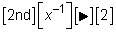to select the Transpose command from the MATRX MATH menu. See the first screen.

• Entering the identity matrix: You don’t have to enter a matrix in order to find the identity matrix. To enter an identity matrix in an expression, press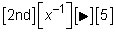to select the identity command from the MATRX MATH menu. Then enter the size of the identity matrix. For example, enter 2 for the 2 x 2 identity matrix, as shown in the second screen.

If you raise a square matrix to the zero power, you get the identity matrix. See the third screen. How cool is that?